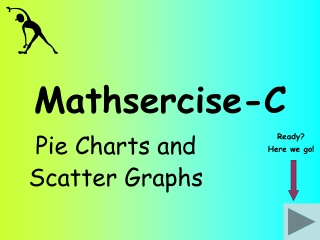DownloadDownload PresentationMathsercise-C

# Mathsercise-C

Télécharger la présentation## Mathsercise-C

- - - - - - - - - - - - - - - - - - - - - - - - - - - E N D - - - - - - - - - - - - - - - - - - - - - - - - - - -
##### Presentation Transcript

1. Mathsercise-C Pie Charts and Scatter Graphs Ready? Here we go!

2. Sixth Form College 120o 60o Don’t Know 1 1 3 3 60 students were asked “What do you want to do next year?” Their replies are shown in the pie chart Question 2 Calculate what proportion of the circle represents college Answer How many students hoped to go to college? 360 ÷ 120 = 1 Pie Charts & Scatter Graphs x 60 = 20 students

3. Forty students took the maths GCSE last year x 9 Grade ‘B’ – 3 Grade ‘C’ – 15 Grade ‘D’ – 14 Grade ‘E’ - 8 27o Question 3 x 9 135o x 9 126o x 9 72o x 9 = 360o 40 Answer If these results were shown in a pie chart, what is the size of angle for each grade? Now work out the multiplier 2 Pie Charts & Scatter Graphs

4. x x x x x x x x x x x x x x x x x x x Here is a scatter graph. One axis is labelled ‘Height’ a) For this graph state the type of correlation Question 4 Positive (going upwards!) Answer b) From the list below, circle the most appropriate label for the other axis GCSE Maths Mark No. of cousins 3 Pie Charts & Scatter Graphs Size of feet Colour of eyes

5. For each scatter graph, sketch in probable correlations x x x x Height x x x x x x x x x x x x x x x x x x x x x x Colour of eyes Start Height Age (up to 10) Answer 4 Pie Charts & Scatter Graphs